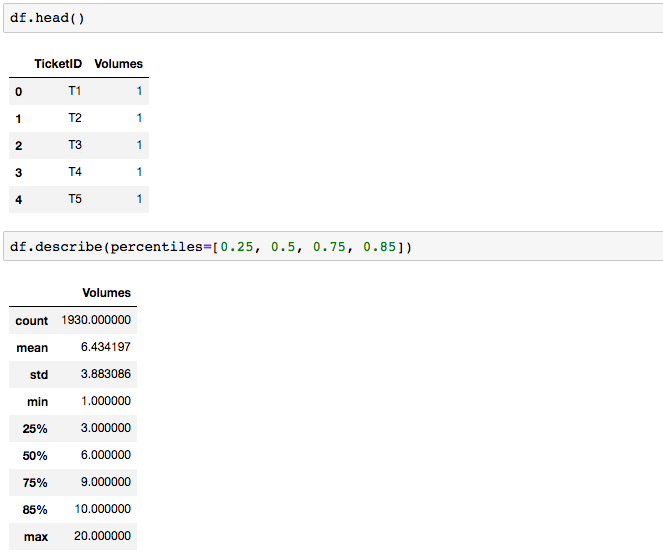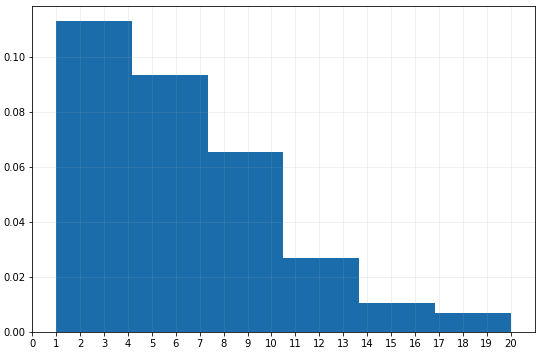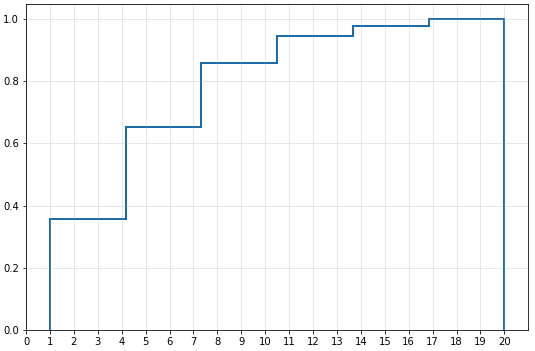# Matplotlib Series 11: Histogram

This blog specifies how to create/custom basic histogram and cumulative histogram with matplotlib in Python and their use cases.

This blog is part of Matplotlib Series:

## Histogram

A histogram is an accurate representation of the distribution of numerical data. It differs from a bar graph, in the sense that a bar graph relates two variables, but a histogram relates only one. To construct a histogram, the first step is to “bin” (or “bucket”) the range of values-that is, divide the entire range of values into a series of intervals—and then count how many values fall into each interval. The bins are usually specified as consecutive, non-overlapping intervals of a variable. The bins (intervals) must be adjacent, and are often (but are not required to be) of equal size.

### When to use it ?

• Estimating the probability distribution of a continuous variable (quantitative variable).
• Organizing large amounts of data, and producing a visualization quickly, using a single dimension.

### Dataframe### Basic histogramThis plot describes that among 1930 tickets, 11% tickets contain less than 5 products; less than 1% tickets contain less than 21 products but more than 16 products. However, if we want to the percentage of tickets that contains less than or egale to 10 products, this basic histogram cannot satisfy our need in one second. In the following cumulative histogram, we can find the answer.

### Cumulative histogramConsidering the same question as above: what the percentage of tickets that contain less than or egale to 10 products? According to this cumulative histogram, the answer is obvious: nearly 85% tickets contain less than or egale to 10 products.